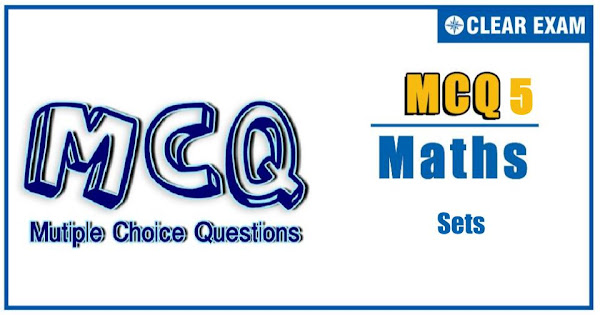## [LATEST]\$type=sticky\$show=home\$rm=0\$va=0\$count=4\$va=0

Mathematics is an important subject in JEE ADVANCED and other engineering exams. In any engineering enterance exam, Mathematics carries weightage of 33% of questions. In mathematics a set is a collection of distinct elements. The elements that make up a set can be letters, numbers, points in space, lines, other geometrical shapes, variables, or even other sets. Two sets are equal if and only if they have precisely the same elements. With focused practice good marks can be fetched from this section. These questions are very important in achieving your success in JEE and Other Engineering Exams.

Q1. In the set A={1,2,3,4,5}, a relation R is defined by R={(x,y):x,y∈A and x<y}. Then, R is
•  Reflexive
•  Symmetric
•  Transitive
•  None of these
Q2.Let n be a fixed positive integer. Define a relation R on the set Z of integers by, a R b⇔n | a-b. Then, R is not
•  Reflexive
•  Symmetric
•  Transitive
•  None of these
Solution
d) None of these
Q3.  Let R and S be two equivalence relations on a set A. Then,
•  R∪S is an equivalence relation on A
•  R∩S is an equivalence relation on A
•  R-S is an equivalence relation on A
•  None of these
Solution
b) R∩S is an equivalence relation on A

Q4. Consider the set A of all determinants of order 3 with entries 0 or 1 only. Let B be the subset of A consisting of all determinants with value 1. Let C be the subset of the set of all determinants with value -1. Then
•  C is empty
•  B has as many elements as C
•  A=B∪C
•  B has twice as many elements as C
Q5.Let L be the set of all straight lines in the Euclidean plane. Two lines l_1 and l_2 are said to be related by the relation R iff l_1 is parallel to l_2. Then, the relation R is not
•  Reflexive
•  Symmetric
•  Transitive
•  None of these
Solution
d) None of these
Q6. In a set of teachers of a school, two teachers are said to be related if they “teach the same subject”, then the relation is
•  Reflexive and symmetric
•  Symmetric and transitive
• Reflexive and transitive
•  Equivalence
Q7.An investigator interviewed 100 students to determine the performance of three drinks milk, coffee and tea. The investigator reported that 10 students take all three drinks milk, coffee and tea; 20 students take milk and coffee, 30 students take coffee and tea, 25 students take mile and tea, 12 students take milk only, 5 students take coffee only and 8 students take tea only. Then, the number of students who did not take any of the three drinks, is
•  10
•  20
•  25
•  30
Q8.The relation R defined in N as a R b⟺b is divisible by a is
•  Reflexive but not symmetric
•  Symmetric but not transitive
•  Symmetric and transitive
•  None of these
Q9.The number of elements in the set {(a,b):2a2+3b2=35,a,b∈Z}, where Z is the set of all integers, is
•  2
•  4
•  8
•  12
Q10. If A={x,y,z}, then the relation R={(x,x),(y,y),(z,z),(z,x),(z,y)} is
•  Reflexive
•  Antisymmetric
•  Transitive
• Both (a) and (b)#### Written by: AUTHORNAME

AUTHORDESCRIPTION## Want to know more

Please fill in the details below:

## Latest NEET Articles\$type=three\$c=3\$author=hide\$comment=hide\$rm=hide\$date=hide\$snippet=hide

Name

ltr
item
BEST NEET COACHING CENTER | BEST IIT JEE COACHING INSTITUTE | BEST NEET, IIT JEE COACHING INSTITUTE: Sets-Quiz-5
Sets-Quiz-5
https://1.bp.blogspot.com/-LZDWsMh0ZEc/YN6KRPXOvTI/AAAAAAAAF84/HR3iRO0iGOEOaFgKQSFKp8vrL1iWaedawCLcBGAsYHQ/s600/Quiz%2BImage%2B5.jpg
https://1.bp.blogspot.com/-LZDWsMh0ZEc/YN6KRPXOvTI/AAAAAAAAF84/HR3iRO0iGOEOaFgKQSFKp8vrL1iWaedawCLcBGAsYHQ/s72-c/Quiz%2BImage%2B5.jpg
BEST NEET COACHING CENTER | BEST IIT JEE COACHING INSTITUTE | BEST NEET, IIT JEE COACHING INSTITUTE
https://www.cleariitmedical.com/2021/07/sets-quiz-5.html
https://www.cleariitmedical.com/
https://www.cleariitmedical.com/
https://www.cleariitmedical.com/2021/07/sets-quiz-5.html
true
7783647550433378923
UTF-8